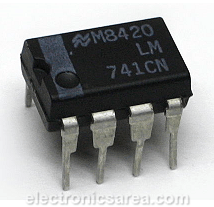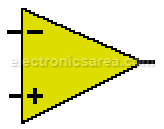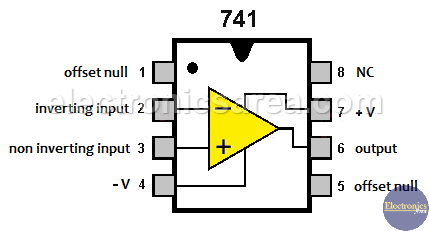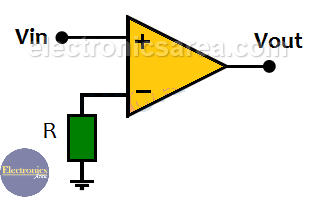# What is an Op Amp? What is an Op Amp Open Loop Gain?

## Brief history of the Operational Amplifier

Op Amp (Op-Amp) stands for operational amplifier. The Operational Amplifier was developed for use in analog computers in the early 1940s. The first Op Amp used vacuum tubes that were very large and very energy consuming.

In 1967 the “Fairchild Semiconductor” company introduced the first operational amplifier in a integrated circuit package, reducing its size, energy consumption and price. This device is a high performance linear amplifier, with a wide variety of uses.

For a more complete history go to History of the Op Amp.## Op Amp Features

Basically Op Amp is a device that amplifies the difference of its two inputs, with a high gain, a very high input impedance, (greater than 1 Mega ohm) and a low output impedance (from 8 to 20 ohms).

With these characteristics it follows that the input currents are practically zero and that it can deliver relatively high output current (see manufacturer data). Op Amp is made of many transistors, resistors, capacitors, etc.Operational Amplifier Symbol

The + terminal is the non-inverting terminal. The terminal – is the inverting terminal. There are several types of presentation of operational amplifiers, such as the 8-pin dual in-line (DIP) package (see the first image).

To know which pin # 1 is, a notch is located between pins 1 and 8, with # 1 being the pin that is to the left of the notch when the integrated circuit is placed as shown in the diagram.

The distribution of the Op Amp pins in the 8-pin DIP integrated circuit is:

• Pin 1 is Offset null.
• Pin 2 is Inverting input (-).
• Pin 3 is a non-inverting input (+).
• Pin 5 is offset null.
• Pin 6 is the output voltage.
• Pin 8 is not connected.IC 741 Op Amp Pinout

To power an operational amplifier, 2 voltage sources are needed:

• Pin 4 is -V  voltage supply (-VCC)
• Pin 7 is +V voltage supply (+VCC)

## Op Amp Open Loop Gain

The Op Amp open loop gain is given when there is no feedback path between the output and one of the two inputs. See the diagram below.

The open loop gain of the amplifier is given by the following formula: AV = Vout/Vinwhere:

• AV = voltage gain
• Vout = output voltage
• Vin = input voltage = (V+ – V)

In an ideal Op Amp open loop gain (operational amplifier the gain) is infinite. Since the op amp is real, its gain is between 20,000 and 200,000 (in 741C operational amplifier). This type of configuration is used in comparators, where you want to know which of the two inputs has the highest voltage.

You may also like: Op amp Voltage follower (buffer)

Note: Op Amp = Op-Amp = Operational Amplifier

•
•
•
•
•
•
•
•
•
•
•
•
•
•
•
•
•
•